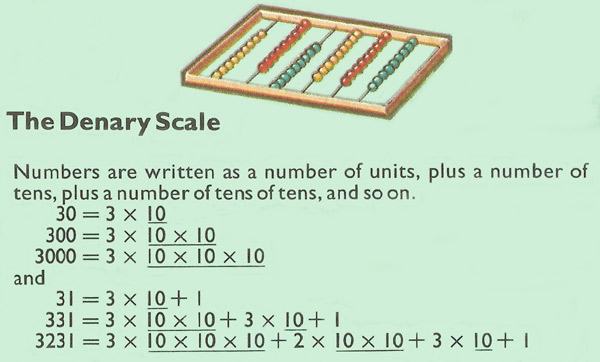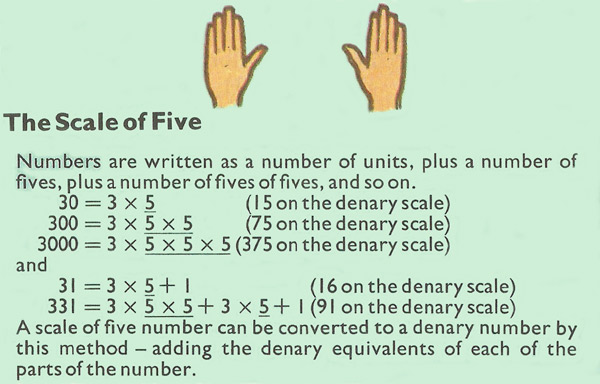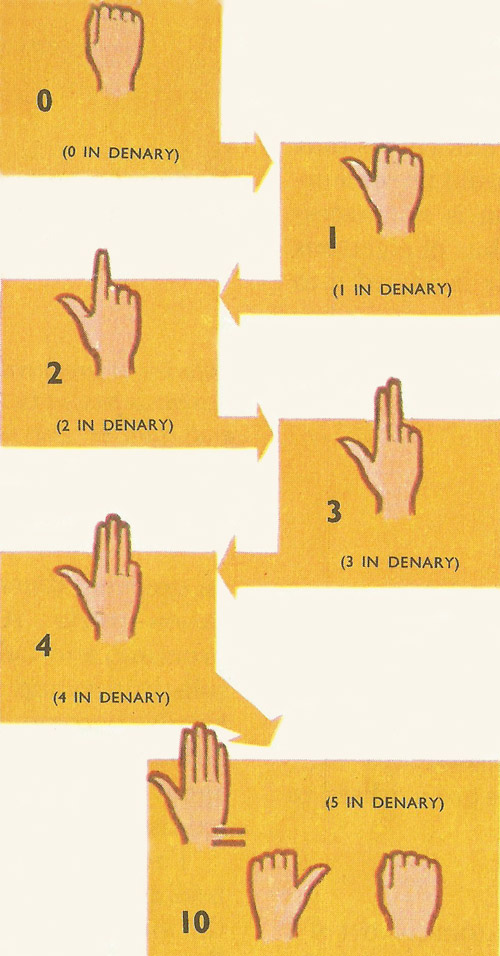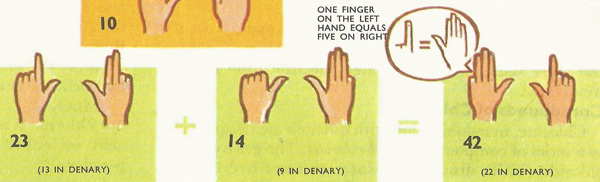A# number systemFig 1. The denary, or decimal, scale.Fig 2. Scale of five.Fig 3. Counting in fives.A number system is a way of counting using a particular base. The familiar decimal, or denary, number system is a base 10 system, because it uses ten different digits, 0, 1, 2, 3, 4, 5, 6, 7, 8, 9, and combinations of these. Other number systems, used for special purposes or by certain cultures, are the binary (base 2), trinary (base 3), duodecimal (base 12), hexadecimal (base 16), vigesimal (base 20), and sexagesimal (base 60).

In a positional number system of base b, b basic symbols (or digits) corresponding to the first b natural numbers, including zero, are used. To generate the rest of the numbers, the position of the symbol in the figure is used. The symbol in the last position has its own value, and as it moves to the left its value is multiplied by b. In this way, with only finitely many different symbols, every number can be expressed. This is unlike systems, such as that of Roman numerals, which use different symbols for different orders of magnitude.

## Denary scale

Our number system is based on counting in tens. There are ten digits, from 0 to 9. After this come the number of tens, and the number of tens of tens (hundreds). 31 is equal to three tens and one unit (Fig 1). 648 is equal to six hundreds plus four tens plus eight units.

31 = 3 tens + 1 unit
648 = 6 hundreds + 4 tens + 8 units.

The 'tens' scale is called the denary scale.

When adding in the denary scale, the units are added together first, then the tens, then the hundreds, and so on. When the number of units comes to 10 or more, extra tens are carried on to the tens column. When the number if tens is ten or more, extra hundreds are carried to the hundreds column.

## Other number systems

Ten is by no means the only basis for a number system. Ancient man used to think in fives because he had five fingers on his hand (the clumsy Roman system is also based, among other things, on fives). There is no number five in a system based on five (Fig 2). Neither are there the digits 6, 7, 8, 9. 5 in this system is written as 10, and 6 as 11. Counting in fives goes:

1, 2, 3, 4, 10, 11, 12, 13, 14, 20...

21 in the 'fives' system does not stand for the same number as 21 in the denary system. 21 in 'fives' corresponds to 11 in 'tens'.

## Binary

No one now uses the fives system, but a great many modern electronic computers think in a 'twos' system. This is called the binary system. There are no symbols 2, 3, 4, 5, 6, 7, 8 or 9. 2 becomes 10. 3 becomes 11. 4 becomes 100 and so on. In fact, all numbers are represented on the binary scale by just two digits, 1 and 0.

 Denary: 1 2 3 4 5 6 7 8 Binary: 1 10 11 100 101 110 111 1000

There is a straightforward way of converting any denary number into a binary number. The denary number is successively divided by 2. Each time there is a or 0).

For example, 7 divided by 2 is 3, remainder 1. The first digit of the binary number (starting from the right) is therefore 1. To find the second digit, 3 is divided by 2. The result is 1, remainder 1. The second digit is 1, and the third digit must also be 1, for there is only a 1 remaining. This method works for all numbers, no matter how large. If an even number (i.e. divisible by 2) appears at any stage, the remainder is 0.